## Math 501: Differential Geometry

Homework 3 <me@tylerlogic.com>

February 10, 2013
http://coursework.tylerlogic.com/courses/math501/homework03

1

2 do Carmo pg 65 exercise 1

The set S is the cylinder {(x,y,z) 3|x2 + y2 = 1}. Define f : 3 by the mapping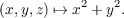With this definition, f-1(1) is exactly S since S is the set of all points of which have x2 + y2 = 1. Since the differential df(x0,y0,z0) is the left multiplication by the matrix (2x0,2y0,0), then the only point critical point of the differential is (0,0,0). Putting the previous two ideas together then gives us that 1 is a regular point of the mapping f since f-1(1) = S doesn’t contain the origin of 3. Hence, because f is smooth, then do Carmo’s Proposition 2 of Section 2-2 informs us that S is regular.

3 do Carmo pg 65 exercise 2

Denote the open disc, {(x,y,z) 3 | z = 0 and x2 + y2 < 1}, by D and the closed disc {(x,y,z) 3 | z = 0 and x2 + y2 1}, by D.

Closed Disc The closed disc D is not a regular surface because if it were, then by the definition of a regular surface there would exist a neighborhood V 3 of (0,1) D such that DV is homeomorphic to some open set of 2, say U. However, the point (0,1) is in D V but is not an interior point, which contradicts the fact that U, being open in 2, contains only interior points.

Open Disc Setting U 2 to the open set {(x,y) 2 | x2 + y2 < 1} and defining f : U by f(u,v) = 0, then D is simply the graph {(u,v,f(u,v)) | (u,v) U} of 3. So D is a regular surface by do Carmo’s first proposition of chapter two.

4 do Carmo pg 66 exercise 11

Let S be the set {(x,y,z) 3 | z = x2 - y2}.

Regular Surface Define f : 3 by f(x,y,z) = x2 -y2 -z. With this definition, f is smooth and dfp for p = (x0,y0,z0) is the linear map corresponding to the matrix (2x0,-2y0,-1). Since the third parameter of the matrix is -1, then dfp is surjective. Since p was arbitrary, we know the differential to be surjective at all points of 3, and thus all points of are regular values of the map f. Hence by do Carmo’s second proposition of section 2-2, f-1(0) is a regular surface, but f-1(0) is all points that satify 0 = x2 - y2 - z, which is exactly the set S. So S is regular.

Parametrizations For the following, note that S can equivalently be given by the equation x2 - y2 - z = 0.

(a)

The parametrization defined by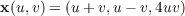covers S due to the following.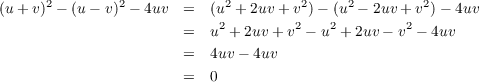(b)

The parametrization defined by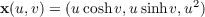covers S due to the following.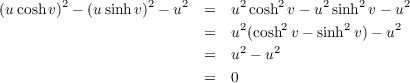Coverings of the parametrizations ???

5 do Carmo pg 67 exercise 16

(a)

For the π-1 definition of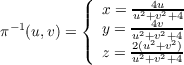Letting d = u2 + v2 for convience we have the following.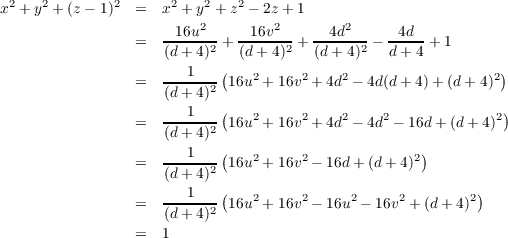(b)

6

The function f(x) = x3 satisfies our needs. It is certainly smooth. It is bijective with inverse f-1(x) =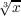and since both it and the inverse are continuous, then f is a homeomorphism. However, the inverse is not differentiable at x = 0 since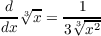and this is undefined at x = 0.

### References

   Rudin, Walter. Principles of Mathematical Analysis, 3rd ed. McGraw-Hill Inc. New York, 1976.